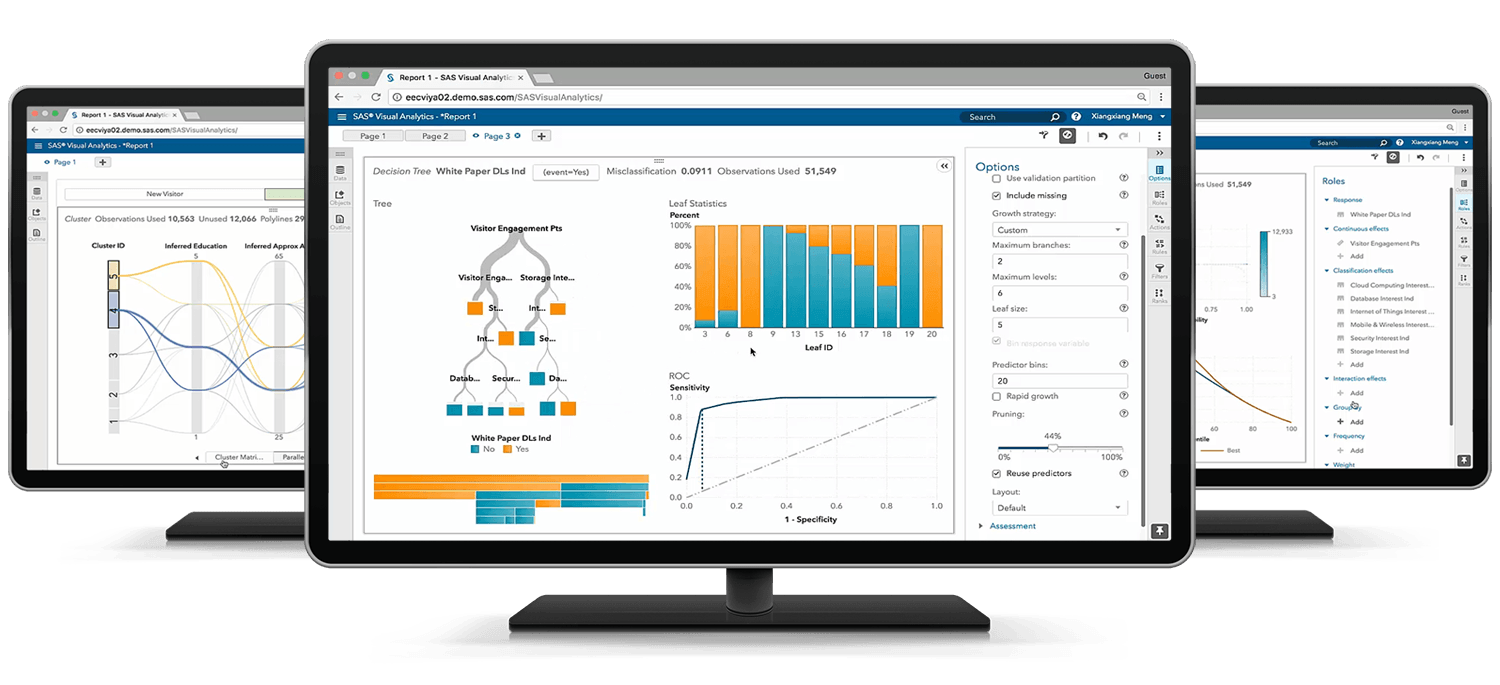SAS Big Data Preparation, Statistics, and Visual Exploration

Exam Content Guide

Below we provide a list of the objectives that will be tested on the exam.Data Management - 50%

• Navigate within the Data Management Studio Interface
• Create, design and be able to explore data explorations and interpret results
• Define and create data collections from exploration results
• Create and explore a data profile
• Design data standardization schemes
• Create Data Jobs
• Apply a Standardization definition and scheme
• Apply Parsing definitions
• Compare and contrast the differences between identification analysis and right fielding nodes
• Apply the Gender Analysis node to determine gender
• Create an Entity Resolution Job
• Define and create business rules
• Describe the organization, structure and basic navigation of the QKB
• Be able to articulate when to use the various components of the QKB
• Define the processing steps and components used in the different definition types

ANOVA and Regression - 30%

• Verify the assumptions of ANOVA
• Analyze differences between population means using the GLM and TTEST procedures
• Perform ANOVA post hoc test to evaluate treatment affect
• Detect and analyze interactions between factors
• Fit a multiple linear regression model using the REG and GLM procedures
• Analyze the output of the REG, PLM, and GLM procedures for multiple linear regression models
• Use the REG or GLMSELECT procedure to perform model selection
• Assess the validity of a given regression model through the use of diagnostic and residual analysis
• Perform logistic regression with the LOGISTIC procedure
• Optimize model performance through input selection
• Interpret the output of the LOGISTIC procedure

Visual Data Exploration - 20%

• Examine, modify, and create data items
• Select and work with data sources
• Create, modify, and interpret automatic chart visualizations in Visual Analytics Explorer
• Create, modify, and interpret graph and table visualizations in Visual Analytics Explorer
• Enhance visualizations with analytics within Visual Analytics Explorer
• Interact with visualizations and explorations within Visual Analytics Explorer